# Partial derivative

(diff) ← Older revision | Latest revision (diff) | Newer revision → (diff)

## Definition at a point

### For a function of two variables

Suppose$f$ is a real-valued function of two variables$x,y$, i.e., the domain of$f$ is a subset of$\R^2$. We define the partial derivatives as follows:

• Partial derivative with respect to$x$: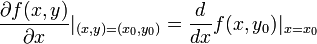$\frac{\partial f(x,y)}{\partial x}|_{(x,y) = (x_0,y_0)} = \frac{d}{dx}f(x,y_0)|_{x = x_0}$

In words, it is the derivative at$x = x_0$ of the function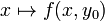$x \mapsto f(x,y_0)$.

This partial derivative is also denoted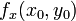$f_x(x_0,y_0)$ or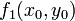$f_1(x_0,y_0)$.

• Partial derivative with respect to$y$: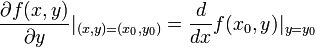$\frac{\partial f(x,y)}{\partial y}|_{(x,y) = (x_0,y_0)} = \frac{d}{dx}f(x_0,y)|_{y = y_0}$

In words, it is the derivative at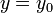$y = y_0$ of the function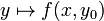$y \mapsto f(x,y_0)$.

This partial derivative is also denoted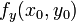$f_y(x_0,y_0)$ or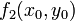$f_2(x_0,y_0)$.

### For a function of multiple variables

Fill this in later